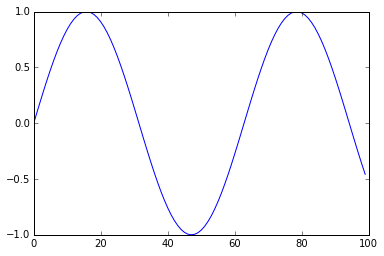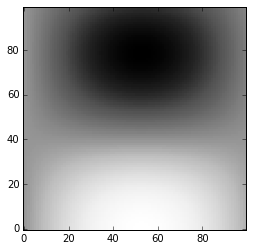# Computational Vision (Psy 5036): Python¶

So far in this course we've tried to emphasize concepts usually with toy examples. We'll now go over tools that can be applied to state-of-the-art problems in computational vision. Mathematica is excellent for learning concepts, and for many high-end applications. However, it is not so good for specialized work in the field where others are using different tools. Matlab and increasingly Python have large user communities who are building tools that we can build on. That's the goal. But first an introduction to IPython as an environment for scientific programming.

The purpose notebook is to provide an overview of the Python/IPython environment with a guide to resources for learning.

## Starting IPython in the middle¶

In order to run this notebook on your computer, you will need to have Python and IPython installed. Find the directory where you've downloaded this notebook, and then from a terminal command line go the directory (or parent directory) and type: ipython notebook. This will bring up a browser window from where you should see this notebook listed in your browser window. You can load it from there.

###### Installation¶

The hardest, or at least the most frustrating, aspect of Python can be installation. There are a number of package managers. We recommend Anaconda.

In addition to Python and IPython, you will need numpy, matplotlib and the scipy library. All of these are part of the scipy stack for general purpose scientific programming.

For image processing, we'll use scikit-image. This, like the machine learning module scikit-learn, is built on the scipy stack.

There is also increasing support for "Open Source Computer Vision OpenCV" on python.

For Bayesian computations using MCMC sampling see pymc.

###### Rationale for scientific python and IPython notebooks¶

See this 2014 article in Nature. For an introduction to IPython.

For scientific python using notebooks, see in-depth series.

### Style¶

Let's first cover some style used in notebooks. Right off the bat, you can create various kinds of cells: Raw NBConvert, various headings, or a Markdown cell. Try double-clicking on some of the cells in this notebook. Code cells are for code.

This cell is Markdown. You can type in LaTeX commands and have them formatted. E.g. try putting the next line between double dollar signs:

p(y_i|x)= \frac{e^{y_i(w.x+b)}}{{1+e^{(w.x+b)}}}

After getting acquainted with the menu items and buttons of the IPython notebook interface, take a look at these notes on: IPython's Rich Display System. Try copying in cell content in this notebook to try out displaying different kinds of content.

### Making and plotting lists¶

In :
import numpy as np


To get started, let's look at some simple python coding examples. We need to load numpy to handle vectors and matrices. To make lists in Mathematica we typically used Table[], e.g.

Table[Sin[x], {x, 0, 1, .1}];



In python, we'll use "list comprehensions". Create a code cell and try these:

[sin(x) for x in arange(0,1,.1)]

[sin(x) for x in linspace(0,1,10)]



But wait! Python needs to know where these functions came from. arange(), linspace(), and sin() are all numpy functions. We imported numpy functions with the shorthand "np", so you will need to write:

np.sin(x), and np. arange(0,1,.1).



For more on creating and manipulating numerical lists in NumPy see scipy page

In [ ]:


In :
sl=[np.sin(x) for x in np.arange(0,10,.1)]


Let's plot these values. To do this, we'll need to import matplotlib, and in particular the pyplot module, or plt for short. If you want a more matlab like plotting environment, you can use pylab:

from pylab import *



For more information on plotting, see scipy notes, and Lecture 4 in the scientific python notebook series.

How to get information about a function? In Mathematica you can type ?Sin. In an IPythoncode cell try typing:?sin. Now try ?np.sin.

In :
import matplotlib.pyplot as plt

In :
plt.plot(sl)
plt.show()With the magic function the next cell, your plot should appear in a separate window. Close the figure window and now excecute the next cell below, and then run the above plot cell again.

In :
%matplotlib inline


We made 2D lists in Mathematica using the Table[] function like this:

Table[Sin[x] Cos[y], {x, 0, 1, .1}, {y, 0, 1, .1}];



Now try using the list comprehension syntax for python:

[[sin(x)*cos(y) for x in arange(0,1,.1)] for y in arange(0,1,.1)] or

[[sin(x)*cos(y) for x in linspace(0,1,10)] for y in linspace(0,1,10)]



Again remember to specify the numpy class using np.

In :
#If you remove the semicolon and it is the last line, the next line will print out the values
[[np.sin(x)*np.cos(y) for x in np.arange(0,1,.1)] for y in np.arange(0,1,.1)];

In :
#but it won't if you assign a variable name to the array

sl2=[[np.sin(x)*np.cos(y) for x in np.arange(0,1,.1)] for y in np.arange(0,1,.1)];

###### Getting parts of vectors and arrays¶
In :
#make a matrix with 5 rows and 6 columns
sl3=np.array([[x+y*6 for x in np.arange(0,6,1)] for y in np.arange(0,5,1)])
#what happens if you don't use np.array() in the above line?

print np.array(sl3),'\n\n(#rows, #columns)=',np.shape(sl3),"# elements:",np.size(sl3)

[[ 0  1  2  3  4  5]
[ 6  7  8  9 10 11]
[12 13 14 15 16 17]
[18 19 20 21 22 23]
[24 25 26 27 28 29]]

(#rows, #columns)= (5, 6) # elements: 30

In :
#note that unlike Mathematica and Matlab (but like C), indexing starts with 0
#first row
sl3

Out:
array([0, 1, 2, 3, 4, 5])
In :
#first column
sl3[:,0]

Out:
array([ 0,  6, 12, 18, 24])
In :
#Try accessing the element in the 3rd row, 4th column. It isn't sl3[3,4]|

#Get the first 2 elements of sl. It isn't sl[0:1].

In :
#Now get the submatrix 3rd and 4th row, 4th through 6th columns
sl3[2:4,3:6]

Out:
array([[15, 16, 17],
[21, 22, 23]])
###### Defining functions¶
In :
def f(x,y):
return([[np.sin(3*x)*np.cos(4*y) for x in np.arange(0,1,.01)] for y in np.arange(0,1,.01)])

In :
#Visualize f(x,y) using imshow()

import matplotlib.cm as cm

n = 10
x = np.linspace(-3,3,4*n)
y = np.linspace(-3,3,3*n)
X, Y = np.meshgrid(x,y)
plt.imshow(f(X,Y),cmap = cm.Greys_r,origin='lower',interpolation='nearest');###### IPython Examples¶

For a directory of IPython notebook examples. E.g. here's a demo of receptive field models

###### Python image processing¶

Look over the examples at the scikit-image site: http://scikit-image.org/docs/dev/auto_examples/

In particular, take a look at the normalized cut method for producing segmentations, and related methods, sometimes referred to as "super-pixels".

Contrast these methods with the edge-detection methods you have learned about.

Here are some demonstrations designed by Weichao Qiu for the chapter by Yuille and Kersten, relevant to this course, some of which are duplicated in individual links on the class web page.

###### Python computer vision demonstrations using OpenCV¶

In the next lecture we cover motion perception and optic flow. We will see there an example of using OpenCV to track feature points.

###### Python neural network resources¶

For python code, look at neurolab. This has several topics that should look familiar.

For python code to simulate spiking neurons, see http://briansimulator.org.

In :
?np.arange

In [ ]: